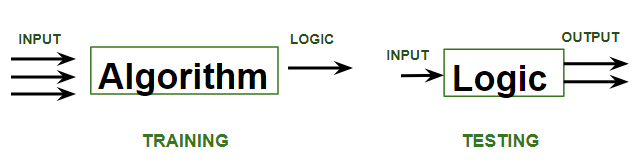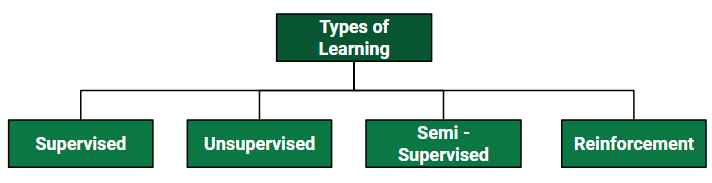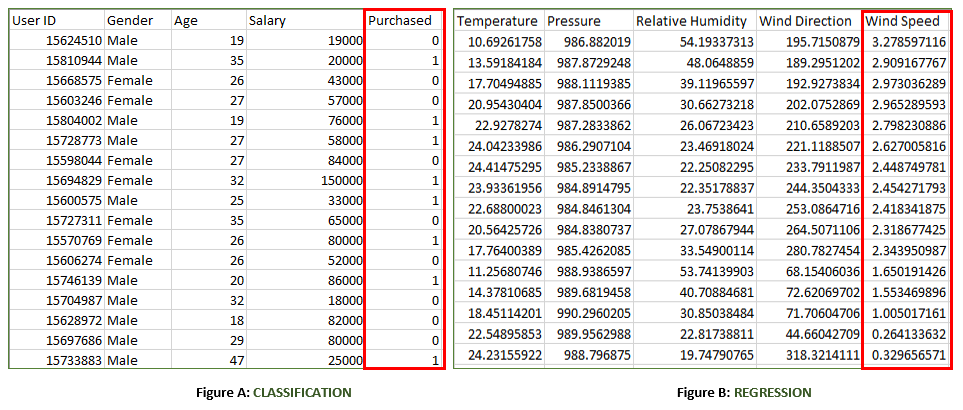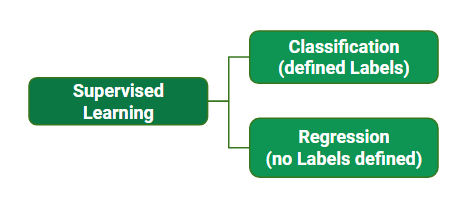Open in App
Not now

# ML | Types of Learning – Supervised Learning

• Difficulty Level : Easy
• Last Updated : 21 Mar, 2023

Let us discuss what is learning for a machine is as shown below media as follows:A machine is said to be learning from past Experiences(data feed-in) with respect to some class of tasks if its Performance in a given Task improves with the Experience. For example, assume that a machine has to predict whether a customer will buy a specific product let’s say “Antivirus” this year or not. The machine will do it by looking at the previous knowledge/past experiences i.e the data of products that the customer had bought every year and if he buys Antivirus every year, then there is a high probability that the customer is going to buy an antivirus this year as well. This is how machine learning works at the basic conceptual level.Supervised learning is when the model is getting trained on a labelled dataset. A labelled dataset is one that has both input and output parameters. In this type of learning both training and validation, datasets are labelled as shown in the figures below.Both the above figures have labelled data set as follows:

• Figure A: It is a dataset of a shopping store that is useful in predicting whether a customer will purchase a particular product under consideration or not based on his/ her gender, age, and salary.
Input: Gender, Age, Salary
Output: Purchased i.e. 0 or 1; 1 means yes the customer will purchase and 0 means that the customer won’t purchase it.
• Figure B: It is a Meteorological dataset that serves the purpose of predicting wind speed based on different parameters.
Input: Dew Point, Temperature, Pressure, Relative Humidity, Wind Direction
Output: Wind Speed

Training the system: While training the model, data is usually split in the ratio of 80:20 i.e. 80% as training data and the rest as testing data. In training data, we feed input as well as output for 80% of data. The model learns from training data only. We use different machine learning algorithms(which we will discuss in detail in the next articles) to build our model. Learning means that the model will build some logic of its own.
Once the model is ready then it is good to be tested. At the time of testing, the input is fed from the remaining 20% of data that the model has never seen before, the model will predict some value and we will compare it with the actual output and calculate the accuracy.### Types of Supervised Learning:

A. Classification: It is a Supervised Learning task where output is having defined labels(discrete value). For example in above Figure A, Output – Purchased has defined labels i.e. 0 or 1; 1 means the customer will purchase, and 0 means that the customer won’t purchase. The goal here is to predict discrete values belonging to a particular class and evaluate them on the basis of accuracy.
It can be either binary or multi-class classification. In binary classification, the model predicts either 0 or 1; yes or no but in the case of multi-class classification, the model predicts more than one class. Example: Gmail classifies mails in more than one class like social, promotions, updates, and forums.

B. Regression: It is a Supervised Learning task where output is having continuous value.
For example in above Figure B, Output – Wind Speed is not having any discrete value but is continuous in a particular range. The goal here is to predict a value as much closer to the actual output value as our model can and then evaluation is done by calculating the error value. The smaller the error the greater the accuracy of our regression model.

Example of Supervised Learning Algorithms:

• Linear Regression
• Logistic Regression
• Nearest Neighbor
• Gaussian Naive Bayes
• Decision Trees
• Support Vector Machine (SVM)
• Random Forest

### ML | Types of Learning – Supervised Learning

• Supervised learning is a type of machine learning in which the algorithm is trained on a labeled dataset, which means that the output (or target) variable is already known. The goal of supervised learning is to learn a function that can accurately predict the output variable based on the input variables. Supervised learning is further divided into two main categories as follows:
1. Classification: In classification, the output variable is a categorical variable, and the goal is to predict the class or category to which a new data point belongs. Examples of classification problems include image classification, spam detection, and sentiment analysis.
2. Regression: In regression, the output variable is a continuous variable, and the goal is to predict the value of the output variable based on the input variables. Examples of regression problems include predicting stock prices, weather forecasting, and sales forecasting.
• Supervised learning algorithms are widely used in various fields, such as natural language processing, computer vision, medical diagnosis, speech recognition, and many others. Some of the popular supervised learning algorithms include: linear regression, logistic regression, decision trees, random forest, k-nearest neighbors (KNN), support vector machine (SVM), and neural networks.
• It’s worth noting that supervised learning is useful when we have a labeled data, and it’s not always the case. In some scenarios, the data is not labeled or it’s too expensive to label, then unsupervised learning, semi-supervised learning, or self-supervised learning could be a better approach.
My Personal Notes arrow_drop_up
Related Articles